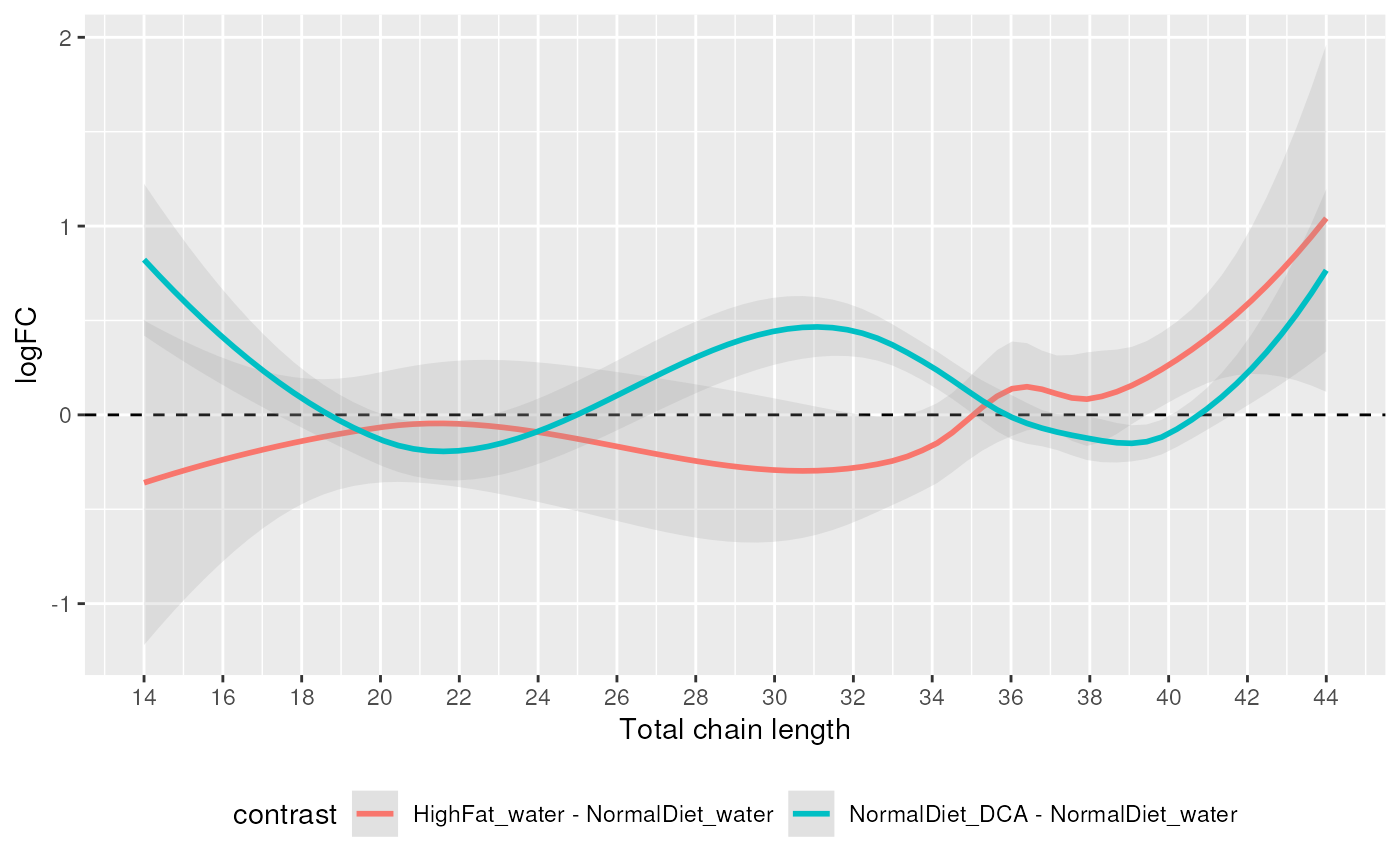Fit and plot a regression line of (log2) fold changes and total chain lengths or unsaturations. If multiple comparisons are included, one regression is plotted for each.

plot_trend(de_results, annotation = c("length", "unsat"))

## Arguments

de_results

Output of de_analysis.

annotation

Whether to fit trend line against chain length or unsat.

A ggplot object.

## Examples

data(data_normalized)
de_results <- de_analysis(
data_normalized,
HighFat_water - NormalDiet_water,
NormalDiet_DCA - NormalDiet_water,
measure = "Area"
)
plot_trend(de_results, "length")
#> geom_smooth() using method = 'loess' and formula 'y ~ x'
#> Warning: Removed 2 rows containing non-finite values (stat_smooth).